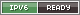### RPGGdx: Yet Another Procedural LandscapeMy first (actual, development-process-related) post here is about a procedural landscape made with LibGDX – the eventual goal being a Daggerfall-like RPG set in a vast procedurally-generated world.

I can bore everyone with half-baked lore ideas later, but for now, let’s figure out how this landscape is made. The first thing I want to do is make some mountainous areas, so we have something interesting to look at.

## Octave Noise

Octave noise is probably nothing new to anyone interested in procedural generation. “Octave Noise” is noise – random data scattered across a 2D plane – which is stretched out and then, generally, overlayed with more noise at smaller scales to create complex patterns.The noise generation itself happens in a few basic steps:

First, we need a function that, for a given set of integers (such as an x and y coordinate and world seed), can output a random number. This is essentially a hash function for the input. This guarantees that a random number generated for some coordinate – say (128, 0) – will always be the same random number. This helps if you’re creating the map in chunks, since you can map the borders of the chunks without having to know what the neighboring chunks have generated.

Some hashes are better than others – low-quality randomness will lead to odd-looking patterns in the landscape. For my purposes, an FNV hash function seems to be decent enough:

public class Noise {
private static final int FNV_PRIME = 0x1000193,
FNV_OFFSETBASIS = 0x811C9DC5;

private static final float INV_2_32 = 1.0f / 4294967296f;

public static float get(int x, int y, int z, long seed) {
long hash = FNV_OFFSETBASIS;

hash = FNV_hash(hash, x);
hash = FNV_hash(hash, y);
hash = FNV_hash(hash, z);
hash = FNV_hash(hash, (int)seed);
hash = FNV_hash(hash, (int)seed >> 32);

hash ^= hash>>32; // xor folding for better randomness
hash &= 0xFFFFFFFF; // slice off top 32 bits

//if (hash*INV_2_32 > 0.5f) return 1; else return 0;
return 0.5f + (int)hash*INV_2_32;
}

private static long FNV_hash(long hash, long value) {
hash ^= (byte)value;
hash *= FNV_PRIME;

hash ^= (byte)value >> 8;
hash *= FNV_PRIME;

hash ^= (byte)value >> 16;
hash *= FNV_PRIME;

hash ^= (byte)value >> 24;
hash *= FNV_PRIME;

return hash;
}
}

We can generate noise based on a given map location, but a landscape needs smooth, rolling hills – the noise needs to be interpolated across many vertices. There are a number of good interpolation strategies that give better results than linear interpolation. Bicubic interpolation is a good one, but I went with trig interpolation:

private static float interpolate(float value1, float value2, float alpha) {
float alpha2 = (1-MathUtils.cos(alpha*MathUtils.PI))/2;
return value1*(1-alpha2) + value2*alpha2;
}

Don’t be fooled by how short the function is. cos() is a fairly expensive call, and you may want to use something else if performance is an issue!

I won’t go through the entire process here – there’s a lot of cruft related to generating the map in regions, and so on – but essentially, using these two functions, you can generate “octaves” of noise by only sampling the noise at certain intervals (say, multiples of 200) and interpolating between them to get smooth curves that are 200 vertices long.

With this, we’re ready to generate the actual landscape data. This is the result from a single 200-vertex-wide octave, copied directly to the heightmap (with height-based coloration to make it more visible, and height ranging from 0 to 500):

heightMap = generate2DFloorOctave(x, z, 200, seed, 0, 500);Well that’s… something.

## Refining the landscape

Of course, the point of octave noise is to layer several octaves of different sizes on top of each other. Here, we take our 200-wide octave, and add some smaller octaves with less weight on top of it:

float[][] data1 = generate2DFloorOctave(x, z, 200, seed, 0, 500);
float[][] data2 = generate2DFloorOctave(x, z, 123, seed+1, 0, 500);
float[][] data3 = generate2DFloorOctave(x, z, 23, seed+2, 0, 500);
float[][] data4 = generate2DFloorOctave(x, z, 7, seed+3, 0, 500);

for (int i = 0; i < Region.WIDTH+1; ++i) {
for (int j = 0; j < Region.WIDTH+1; ++j) {
float h = data1[i][j]*0.7f + data2[i][j]*0.22f + data3[i][j]*0.06f + data4[i][j]*0.02f;

heightMap[i][j] = h;
}
}Getting better! But, if we want something like a mountainous area, we need ridges that this kind of “bumpy” terrain can’t create. But you can create mountain ranges from “bumpy” terrain using an absolute value function:

float[][] data1 = generate2DFloorOctave(x, z, 200, seed, 0, 500);
float[][] data2 = generate2DFloorOctave(x, z, 123, seed+1, 0, 500);
float[][] data3 = generate2DFloorOctave(x, z, 23, seed+2, 0, 500);
float[][] data4 = generate2DFloorOctave(x, z, 7, seed+3, 0, 500);

for (int i = 0; i < Region.WIDTH+1; ++i) {
for (int j = 0; j < Region.WIDTH+1; ++j) {
float h = data1[i][j]*0.7f + data2[i][j]*0.22f + data3[i][j]*0.06f + data4[i][j]*0.02f;

heightMap[i][j] = 500 – Math.abs(h-250)*2;
}
}Here, we’ve taken the middle of the height range, translated the height so that point is at 0 (“h-250”), used Math.abs to create the sharp ridges there, then inverted and raised the result back up (by subtracting it from 500 – so the ridges point up, like a mountain range).We can multiply the result by an even larger octave, to vary the height of the mountain ridges and flatten the land out completely in some areas:

float[][] data0 = generate2DFloorOctave(x, z, 500, seed, 0.1f, 1);
float[][] data1 = generate2DFloorOctave(x, z, 200, seed, 0, 500);
float[][] data2 = generate2DFloorOctave(x, z, 123, seed+1, 0, 500);
float[][] data3 = generate2DFloorOctave(x, z, 23, seed+2, 0, 500);
float[][] data4 = generate2DFloorOctave(x, z, 7, seed+3, 0, 500);

for (int i = 0; i < Region.WIDTH+1; ++i) {
for (int j = 0; j < Region.WIDTH+1; ++j) {
float h = data1[i][j]*0.7f + data2[i][j]*0.22f + data3[i][j]*0.06f + data4[i][j]*0.02f;

heightMap[i][j] = 500 – Math.abs(h-250)*2;

heightMap[i][j] *= data0[i][j];
}
}And with that, we have a semi-decent procedural heightmap for a mountainous terrain. This will basically be a springboard for more interesting topics, like using vertex painting to texture the landscape.